Stephen Dawson
stephendawson@idmail.com
Mathematics 309
Topics in Geometry
Term Project, Spring 2001

The Reflective Property of an Ellipse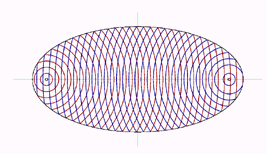The Reflective property of an ellipse is simply this: when a ray leaves one of the foci and meets a point on that ellipse, it will reflect off of the ellipse and pass through the other focus. Now, apart from being mathematically interesting, what makes this property so fascinating? Well, there are several reasons. Most notable of which is its significance to physics, primarily optics and acoustics. Both light and sound are affected in this way. In fact there are many famous buildings designed to exploit this property. Such buildings are referred to as whisper galleries or whisper chambers. St. Paul's Cathedral in London, England was designed by architect and mathematician Sir Christopher Wren and contains one such whisper gallery. The effect that such a room creates is that if one person is standing at one of the foci, a person standing at the other focus can hear even the slightest whisper spoken by the other.

Constructing an ellipse
Finding the tangent to a point on an ellipse
Reflection off of a curve
Reflection off of an ellipse
Why are the angles equal?
Equal angles
Some other constructions

To view the files on this page you may need to obtain a copy of Ghostscript and GSview.
Copies of these can be found here: http://www.cs.wisc.edu/~ghost

Constructing an ellipse

An ellipse can be defined as the locus of points such that the sum of the distances from two fixed points to a point on the ellipse is constant. These two fixed points are called the foci. Based on this definition one can construct an ellipse using a piece of string, something to hold the string in place with and a pencil. The following is a construction of an ellipse using this method (of course in this case the string is made out of pixels on your computer screen). The string is divided into ten equal lengths as to give a sense that the length of the "string" is indeed constant.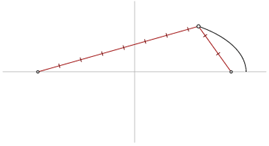Finding the tangent to a point on an ellipse

The following is a series of pictures which show how one goes about finding the tangent to an ellipse, or any curve for that matter. This is done by taking two points, one on either side of the point at which the tangent is to be drawn. A line between them is drawn, and then the points are gradually moved closer and closer until they meet at the desired point. The line that is drawn is called the secant line and it eventually becomes the tangent line. This will be needed later in the discussion.Reflection off of a curve

When an incident ray meets the surface of a curve, the angle it makes with the tangent to that point is equal to the angle between the reflected ray and the tangent.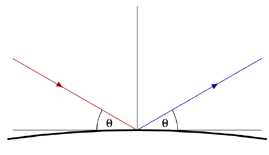Reflection off of an ellipse

The reflective property of an ellipse was mentioned in the introduction, but to reiterate, it occurs when a ray emanates from one of the foci and meets a point on the ellipse. The ray will then reflect off of the tangent to the ellipse, at that point and pass through the other focus. The file on the left shows this very thing. The tangent is found as it was above and the angle between the tangent and incident ray is equal to the angle between the tangent and the reflected ray.

Note: In the picture on the right one cannot distinguish between the tangent line and the ellipse itself. This is because the picture is zoomed in so much that the tangent line is actually flush with the curve at that point.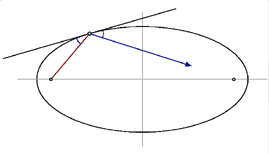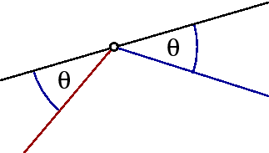Why are the angles equal?

Well, the short answer is that the blue ray is a reflection and so by the definition above, the angles are equal. But this leads to the question: how do we know that the reflected ray passes through the second focus? To answer this question we instead, assume that the ray does pass through the focus and then demonstrate that the angles are equal, thereby showing that the reflected ray passes through the second focus.

To do this we take a second point on the ellipse, move it infinitesimally close to the first point and zoom in extremely close to these points. The file on the left shows this. The file on the right demonstrates why the angles are equal.

For a quick refresher of some basic geometry that is used below click here.Equal angles

The following shows the reflections at all points on the ellipse. Although the angles between the focal lengths and the tangent change as the point moves around the ellipse, the angles nevertheless remain equal to each other.Sound emanating from a focus in any direction will always travel along the focal lengths. And since the sum of these focal lengths is constant, it will always travels the same total distance. So, regardless of direction, the ray takes the same amount of time to leave one focus, reflect off of the ellipse and pass through the other focus (since the velocity of sound is the same). So when sound is emitted radially the reflections arrive at the other focus at the same time.

Both of the following files demonstrate this property. The file on the left shows circles centered at one of the foci reflecting off of the ellipse as circles centered at the other focus. The file on the right shows rays emanating radially which reflect off of the ellipse in the same way shown above. Notice how the reflected rays form a circle around the second focus, just as they do in the file on the left.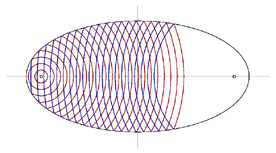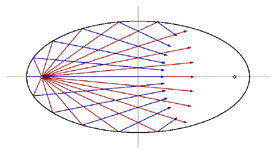Some other constructions

In doing research for this project I came across several ways to construct an ellipse besides the one used above. The following are what I found to be two of the more interesting ways of constructing an ellipse. The one on the left uses concentric circles and with a quick inspection one can see that the radii of the red and blue circles are nothing more than the semi major and minor axis of the ellipse. The one on the right uses intersecting circles to map the points on the ellipse. This construction is essentially the same as the one above, as the two radii of the intersecting circles are equal to the two focal distances with their centers at the two foci. As in the construction above, (where the sum of the focal distances was constant) here the sum of the two radii is constant.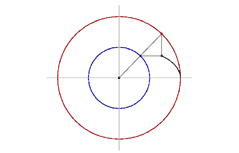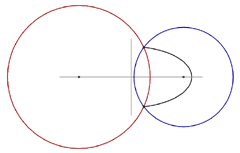To see the original and interactive versions of these and other constructions
go here: Geometry in Motion by Daniel Scher.